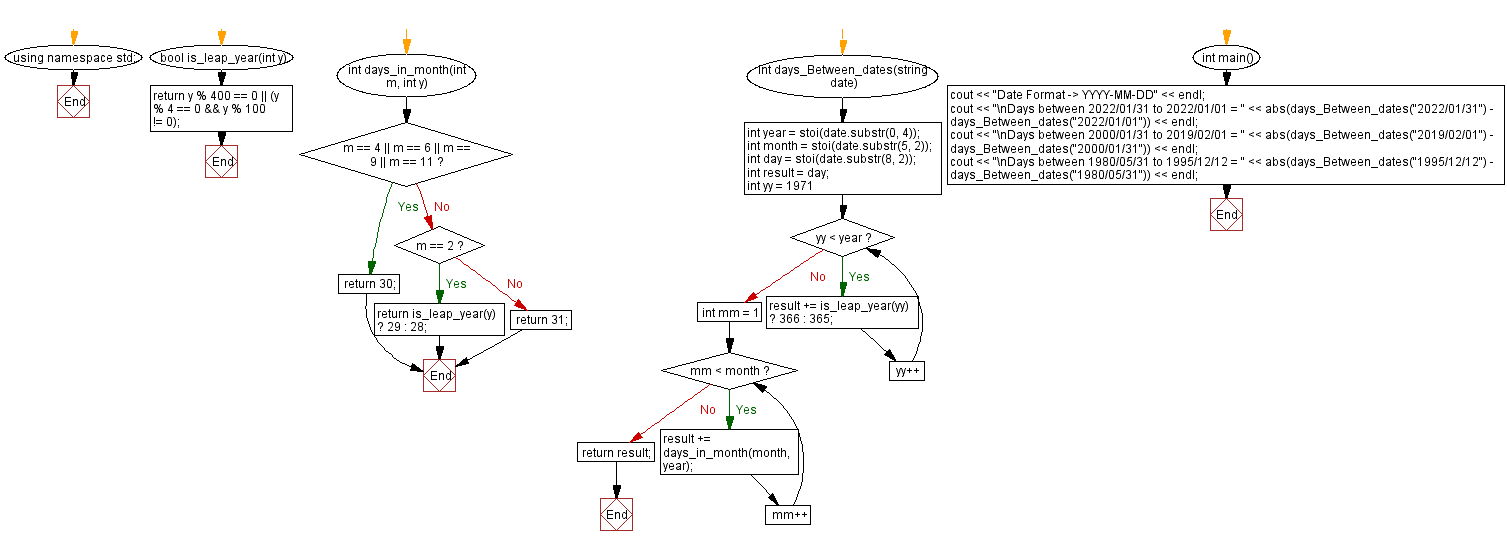﻿ C++ : Count the number of days between two given dates

# C++ Exercises: Count the number of days between two given dates

## C++ Date: Exercise-6 with Solution

Write a C++ program to count the number of days between two given dates.

Sample Solution:

C++ Code:

``````#include <iostream>

#include <string>
using namespace std;
bool is_leap_year(int y) {
return y % 400 == 0 || (y % 4 == 0 && y % 100 != 0);
}
int days_in_month(int m, int y) {
if (m == 4 || m == 6 || m == 9 || m == 11)
return 30;
else if (m == 2)
return is_leap_year(y) ? 29 : 28;
else
return 31;
}

int days_Between_dates(string date) {

int year = stoi(date.substr(0, 4));
int month = stoi(date.substr(5, 2));
int day = stoi(date.substr(8, 2));

int result = day;
for (int yy = 1971; yy < year; yy++)
result += is_leap_year(yy) ? 366 : 365;
for (int mm = 1; mm < month; mm++)
result += days_in_month(month, year);
return result;
}
int main() {
cout << "Date Format -> YYYY-MM-DD" << endl;
cout << "\nDays between 2022/01/31 to 2022/01/01 = " << abs(days_Between_dates("2022/01/31") - days_Between_dates("2022/01/01")) << endl;
cout << "\nDays between 2000/01/31 to 2019/02/01 = " << abs(days_Between_dates("2019/02/01") - days_Between_dates("2000/01/31")) << endl;
cout << "\nDays between 1980/05/31 to 1995/12/12 = " << abs(days_Between_dates("1995/12/12") - days_Between_dates("1980/05/31")) << endl;
}
``````

Sample Output:

```Date Format -> YYYY-MM-DD

Days between 2022/01/31 to 2022/01/01 = 30

Days between 2000/01/31 to 2019/02/01 = 6938

Days between 1980/05/31 to 1995/12/12 = 5677
```

Flowchart:C++ Code Editor: